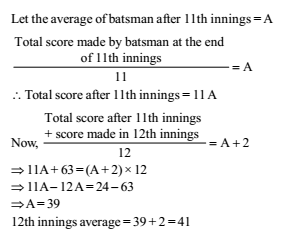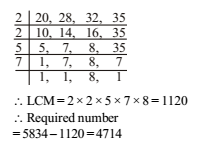# RRB ALP 2018 Practice Test Papers | Arithmetic Questions (Day-41)

Dear Aspirants, Here we have given the Important RRB ALP & Technicians Exam 2018 Practice Test Papers. Candidates those who are preparing for RRB ALP 2018 can practice these Arithmetic Questions to get more confidence to Crack RRB 2018 Examination.

[WpProQuiz 2189]

Click “Start Quiz” to attend these Questions and view Explanation

1. The length and breadth of a square are increased by 30% and 20% respectively. The area of the rectangle so formed exceeds the area of the square by:

(a) 46%

(b) 66%

(c) 42%

(d) 56%

2.A can do a piece of work in 20 days which B can do in 12 days. B worked at it for 9 days. A can finish the remaining work in:

(a) 5 days

(b) 7 days

(c) 11 days

(d) 3 days

3.A batsman in his 12th innings makes a score of 63 runs and there by increases his average scores by 2. What is his average after the 12th innings?

(a) 13

(b) 41

(c) 49

(d) 87

1. A man sold two articles at Rs. 375 each. On one, he gains 25% and on the other, he loses 25%. The gain or loss% on the whole transaction is:

(a) 6%

(b) 41/6 %

(c) Rs. 50

(d) 6  ¼ %

1. A team played 40 games in a season and won in 24 of them. What percent of games played did the team win?

(a) 70%

(b) 40%

(c) 60%

(d) 35%

1. The H.C.F. and L.C.M. of two numebrs are 8 and 48 respectively. If one of the numbers is 24, then the other number is

(a) 48

(b) 36

(c) 24

(d) 16

1. The greatest number, which when subtracted from 5834, gives a number exactly divisible by each of 20, 28, 32 and 35, is

(a) 1120

(b) 4714

(c) 5200

(d) 5600

1. In a family, the average age of a father and a mother is 35 years. The average age of the father, mother and their only son is 27 years. What is the age of the son?

(a) 12 years

(b) 11 years

(c) 10.5 years

(d) 10 years

1. If A’s income is 50% less than that of B’s, then B’s income is what per cent more than that of A?

(a) 125

(b) 100

(c) 75

(d) 50

1. If the ratio of cost price and selling price of an article be as 10: 11, the percentage of profit is

(a) 8

(b) 10

(c) 11

(d) 15

Let the side of square = ‘x’

Area of square = x2

New length of rectangle = 130 x 100

New Breadth of rectangle = 120 x 100

Hence, Area of so formed rectangle = 130/100x 120/100x X2 = 156 x2/ 100

Therefore, area of rectangle exceeds the area of square by 56%

B’s 1 day work = 1 /12

B’s 9 day’s work = 9 /12 =3/4

Remaining work =1- 3 /4=1/ 4

A can finish this work in 20 /4 days = 5 daysIn such type of question, Required% loss = ( 25)2/ 100 %

= 625/100 %

= 6.25%

=6 1/ 4 %

Required percentage = 24/40x 100= 60%

p × q = HCF × LCM

24x q= 8×48

Second number 8x 48/24 = 16Father + mother = 2 × 35 = 70 years

Father + mother + son = 27 × 3 = 81 years

Son’s age = 81 – 70 = 11 years

Required precentage 50/( 100- 50) x100 = 100%

Gain = 11x – 10x = Rs.x

P%= px100/px100 =x/10x *100= 10

RRB ALP 2018 – “All in One” Study Materials and Practice Sets